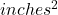Question

If a rectangular prism has a length of 3 1/2 in and a width of 9 in and a height of 3 1/2, what would the surface area be?

1.thuhuong

The surface area of this rectangular prism is 150.5.

Step-by-step explanation:

The formula for finding the surface area of a rectangular prism is this :

A=2(wl + hl + hw)

l = 3.5, w = 9, h = 3.5

Now we substitute those values in and solve for A.

A = 2 · ( 9 · 3.5 + 3.5 · 3.5 + 3.5 ·9) = 150.5

The surface area of this rectangular prism is 150.5.

Hope this helps, please mark brainliest. Have a great day!

2.The surface area is 150.5 in